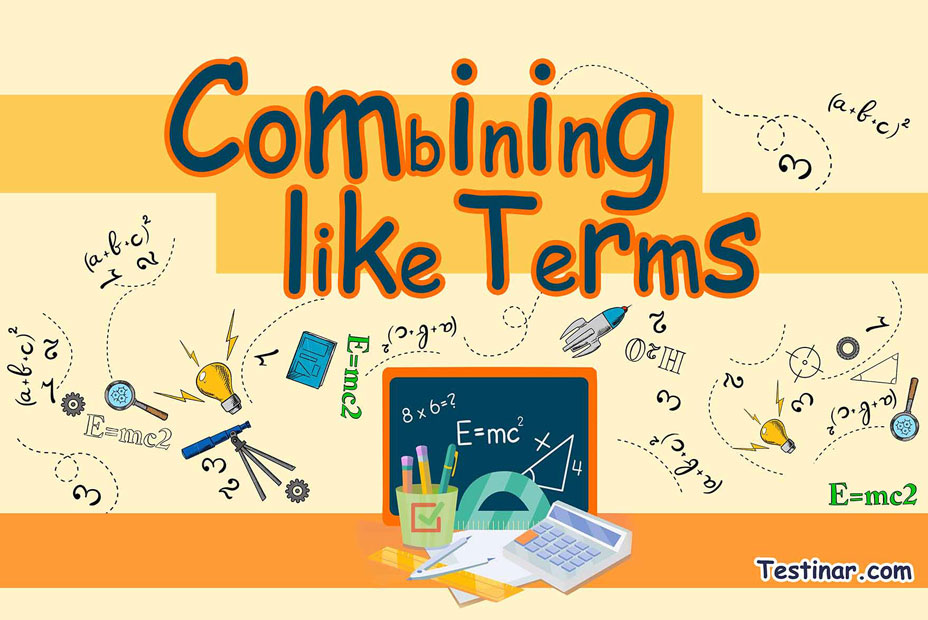## How to Combine Like Terms?

So, let’s understand the meaning of a variable first. We can define a variable as an entity that does not have a fixed value. Suppose there is a variable $$n$$ which is a set of natural numbers. So, $$n$$ belongs to the set of integers ($$1, \ 2, \ 3,$$ ……….). As you can see that $$n$$ can take any values ranging from $$1$$ to infinity. So, $$n$$ is a variable. In mathematics, you will see the use of variables in any alphabet like $$a, \ b, \ x, \ y, \ z, \ m,$$ etc.
Now, since you have understood the term variable, let’s learn about a constant. As the name suggests, a constant is a term that has a fixed value. For example, $$5, \ 6, \ 0.3, \ \frac{3}{5}$$ are all constants.
Now, in mathematics, a term can be either completely constant or completely a variable, or the mixture of the two. For example, let’s take the term $$2z$$. Here $$z$$ is the variable and of course, $$2$$ is the constant term. Also, $$2$$ is called the coefficient of $$z$$. Some other examples of such terms are $$-3y, \ 8x, \ \frac{2}{9} \ a,$$ etc.
Variables, constants, and their mixtures are all identified as terms in mathematics.

### Like and Unlike Terms

In algebra, we have the concept of like and unlike terms. So, if in an expression, you find that two or more same variables have the same exponential powers, then they are considered like terms. So, what we can do to simplify the expression is club those like terms by performing basic mathematical operations between them.
Also, in the case of unlike terms, you will find that the exponential powers of two or more same variables are not the same. So, we have no other option other than to leave them as they are.
Some examples of like terms are $$2x^3 \ - \ x^3, \ 7x^2 \ + \ 3x^2$$. Here we can see that the power of $$x$$ is the same in both the examples. Also, some examples of unlike term expressions would be $$7x^2 \ - \ 9x^3, \ -x^3 \ + \ x^2$$.

### Combining Like Terms

To combine like terms in variable expressions, we must use 2 techniques:

• Firstly, we must add or subtract like terms like those with the same variable (like $$3x, \ -7x$$) or those with the same powers (like $$2x^2, \ -3x^2$$). Also, we must use the same sign for coefficients after combining the like terms.
• Next, we must apply distributive law if possible. The distributive property states that multiplication distributes over addition, i.e., $$x(y \ + \ z) \ = \ (xy \ + \ xz)$$.
Example: $$2x^2(7x \ + \ 9) \ + \ x^2 \ = \ 2x^2(7x) \ + \ 2x^2(9) \ + \ x^2 \ = \ 14x^3 \ + \ 18x^2 \ + \ x^2 \ = \ 14x^3 \ + \ 19x^2$$

### Exercises for Combining like Terms

1) $$7x \ - \ 3x =$$

2) $$20x \ + \ (-3)(3x \ - \ 3 \ + \ x) =$$

3) $$16x \ + \ 2(5x \ - \ 2 \ + \ x) =$$

4) $$21x \ + \ (3x \ - \ 4 \ - \ x) =$$

5) $$5x \ + \ (-2)(4x \ - \ 2 \ + \ x) =$$

6) $$12x \ + \ 2x \ - \ 2 =$$

7) $$5x \ + \ 3x \ - \ 2 =$$

8) $$14x \ + \ (2x \ - \ 4 \ - \ x) =$$

9) $$19x \ + \ 5x \ - \ 2 =$$

10) $$7x \ + \ (5x \ - \ 2 \ - \ x) =$$

1) $$7x \ - \ 3x =$$$$\ \color{red}{4x }$$
2) $$20x \ + \ (-3)(3x \ - \ 3 \ + \ x) =$$$$\ \color{red}{8x \ + \ 9}$$
3) $$16x \ + \ 2(5x \ - \ 2 \ + \ x) =$$$$\ \color{red}{28x \ - \ 4}$$
4) $$21x \ + \ (3x \ - \ 4 \ - \ x) =$$$$\ \color{red}{23x \ - \ 4}$$
5) $$5x \ + \ (-2)(4x \ - \ 2 \ + \ x) =$$$$\ \color{red}{-5x \ + \ 4}$$
6) $$12x \ + \ 2x \ - \ 2 =$$$$\ \color{red}{14x \ - \ 2}$$
7) $$5x \ + \ 3x \ - \ 2 =$$$$\ \color{red}{8x \ - \ 2}$$
8) $$14x \ + \ (2x \ - \ 4 \ - \ x) =$$$$\ \color{red}{15x \ - \ 4}$$
9) $$19x \ + \ 5x \ - \ 2 =$$$$\ \color{red}{24x \ - \ 2}$$
10) $$7x \ + \ (5x \ - \ 2 \ - \ x) =$$$$\ \color{red}{11x \ - \ 2}$$

## Combining like Terms Practice Quiz

### The Most Comprehensive Praxis Core Math Preparation Bundle

$76.99$36.99

### The Most Comprehensive CLEP College Math Preparation Bundle

$76.99$36.99

### The Most Comprehensive ALEKS Math Preparation Bundle

$76.99$34.99

### ISEE Middle Level Math

$16.99$11.99